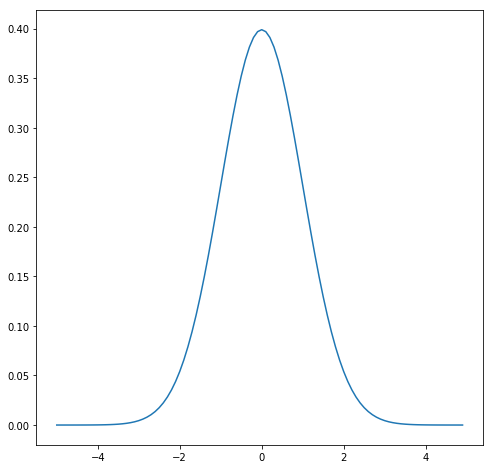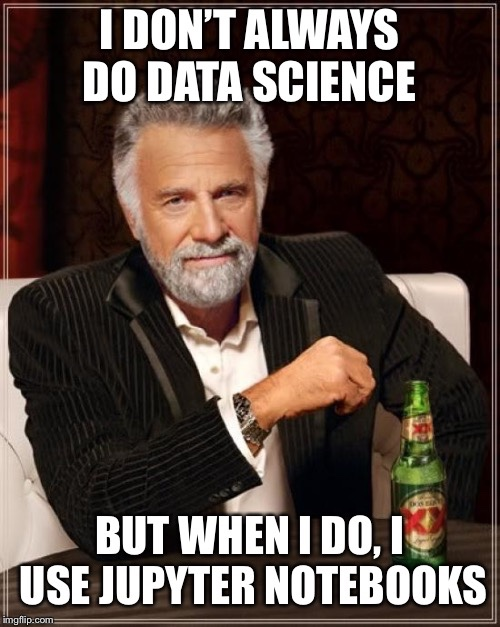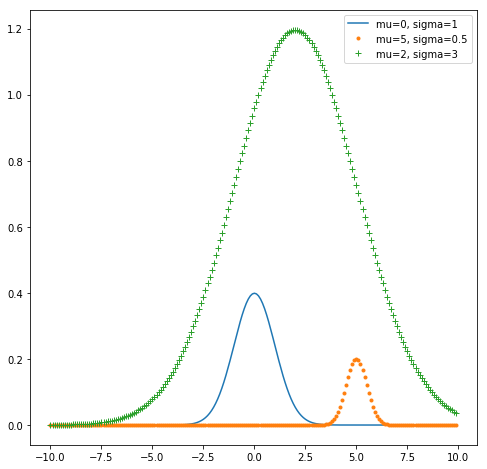## Lesson 3: Support Vector Machines

The term Support Vector Machines or SVM is a bit misleading. It is just a name for a very clever algorithm invented by two Russians. in the 1960s. SVMs are used for classification and regression.

SVM do that by finding a hyperplane between two classes of data which separates both classes best. Continue reading “Lesson 3: Support Vector Machines”

## Linear Algebra with numpy – Part 1

Numpy is a package for scientific computing in Python.

`import numpy as np`

The most important data structure is ndarray, which is short for n-dimensional array.

You can convert a list to an numpy array with the array-method

```my_list = [1, 2, 3, 4]
my_array = np.array(my_list)```

You can also convert an array back to a list with Continue reading “Linear Algebra with numpy – Part 1”

## The Normal Distribution

Diving deeper into data science I started to brush up my knowledge about math especially statistics.

## The Mother of all DistributionsThe normal distribution was formulated by Carl Friedrich Gauß in 18XX and can be implemented in Python like the following :

```def normal_distribution(x, mu=0, sigma=1):
sqrt_two_pi = math.sqrt(2*math.pi)
return math.exp(-(x-mu)**2 / 2 / sigma**2) / sqrt_two_pi * sigma```

## Data Science: Cross-Validation

For validating your model You need to split your data into a training and a test data set.

More training data means a better model, more test data means better validation.

But because the amount of data to train/test the model is limited you have to decide in which ratio of training vs test data you want to split your data.

```import numpy as np
from sklearn.model_selection import train_test_split
from sklearn import datasets
from sklearn import svm

iris.data.shape, iris.target.shape```

Sample a training set while holding out 40% of the data for testing:

`X_train, X_test, y_train, y_test = train_test_split( iris.data, iris.target, test_size=0.4, random_state=0)`
```from sklearn.model_selection import cross_val_score
clf = svm.SVC(kernel='linear', C=1)
scores = cross_val_score(clf, iris.data, iris.target, cv=5)```

Five Minutes with Ingo: Cross Validation

##Do You know the feeling of being already late to a party when encountering something new?

But when you actually start telling others about it, You realize that it is not too common sense at all, e.g. Jupyter Notebooks.

What is a Jupyter notebook? Continue reading “Introduction to Jupyter Notebook”

## Data Science Datasets: Iris flower data set

The Iris flower data set or Fisher’s Iris data set became a typical test case for many statistical classification techniques in machine learning such as support vector machines.

It is sometimes called Anderson’s Iris data set because Edgar Anderson collected the data to quantify the morphological variation of Iris flowers of three related species.

This data set can be imported from scikit-learn like the following:

```from sklearn import datasets

iris.data.shape, iris.target.shape```

## Data Science Overview

Data Science tries to answer one of the following questions:

• Classification -> “Is it A or B?”
• Clustering -> “Are there groups which belong together?”
• Regression -> “How will it develop in the future?”
• Association -> “What is happening very often together?”

There are two ways to tackle these problem domains with machine learning:

1. Supervised Learning
2. Unsupervised Learning

Supervised Learning

You have training and test data with labels. Labels tell You to which e.g. class a certain data item belongs. Image you have images of pets and the labels are the name of the pets.

Unsupervised Learning

Your data doesn’t have labels. Your algorithm e.g. k-means clustering need to figure out a structure given only the data

## The Essence of Machine Learning

The Essence of Machine Learning

1. A pattern exists
2. The pattern cannot be described mathematically
3. We have data on this problem

## My personal road map for learning data science in 2018

I got confused by all the buzzwords: data science, machine learning, deep learning, neural nets, artificial intelligence, big data, and so on and so on.As an engineer I like to put some structure to the chaos. Inspired by Roadmap: How to Learn Machine Learning in 6 Months and Tetiana Ivanova – How to become a Data Scientist in 6 months a hacker’s approach to career planning I build my own learning road map for this year: Continue reading “My personal road map for learning data science in 2018”

## Bayes’ Theorem

Imagine that you come home from a party and you are stopped by the police. They ask you to take a drug test and you accept. The test result is positive. You are guilty.

But wait a minute! Is it really that simple?